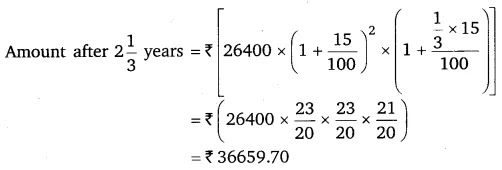NCERT Solutions for Class 8 Maths Chapter 8 Comparing Quantities Ex 8.3

# NCERT Solutions for Class 8 Maths Chapter 8 Comparing Quantities Ex 8.3

## NCERT Solutions for Class 8 Maths Chapter 8 Comparing Quantities Ex 8.3

NCERT Solutions for Class 8 Maths Chapter 8 Comparing Quantities Ex 8.3 are the part of NCERT Solutions for Class 8 Maths. Here you can find the NCERT Solutions for Class 8 Maths Chapter 8 Comparing Quantities Ex 8.3.

### Ex 8.3 Class 8 Maths Question 1.

Calculate the amount and compound interest on
(a) ₹ 10,800 for 3 years at 121/2 % per annum compounded annually.
(b) ₹ 18,000 for 21/2 years at 10% per annum compounded annually.
(c) ₹ 62,500 for 11/2 years at 8% per annum compounded half yearly.
(d) ₹ 8,000 for 1 year at 9% per annum compounded half yearly. (You could use the year by year calculation using SI formula to verify).
(e) ₹ 10,000 for 1 year at 8% per annum compounded half yearly.

Solution:

### Ex 8.3 Class 8 Maths Question 2.

Kamala borrowed ₹ 26,400 from a Bank to buy a scooter at a rate of 15% p.a. compounded yearly. What amount will she pay at the end of 2 years and 4 months to clear the loan?
[Hint: Find A for 2 years with interest is compounded yearly and then find SI on the 2nd year amount for 4/12 years)

Solution:
Here, P = ₹ 26,400, R = 15% p.a. and n = 2 years 4 months =21/3 years.Hence, Kamala will pay ₹ 36659.70 to the bank to clear the loan.

### Ex 8.3 Class 8 Maths Question 3.

Fabina borrows ₹ 12,500 at 12% per annum for 3 years at simple interest and Radha borrows the same amount for the same time period at 10% per annum, compounded annually. Who pays more interest and by how much?

Solution:
In case of Fabina:
P = ₹ 12,500, R = 12% p.a. and T = 3 years. Then,

Hence, Fabina pays ₹ (4500 – 4137.50), i.e., ₹ 362.50 more as interest.

### Ex 8.3 Class 8 Maths Question 4.

I borrowed ₹ 12,000 from Jamshed at 6% per annum simple interest for 2 years. Had I borrowed this sum at 6% per annum compound interest, what extra amount would I have to pay?

Solution:
Here, P = ₹ 12,000, R = 6% p.a. and T = 2 years.

So, I have to pay ₹ (1483.20 – 1440), i.e., ₹ 43.20 extra.

### Ex 8.3 Class 8 Maths Question 5.

Vasudevan invested ₹ 60,000 at an interest rate of 12% per annum compounded half-yearly. What amount would he get
(i) after 6 months?
(ii) after 1 year.

Solution:
Here, P = ₹ 60,000, R = 12% p.a. = 6% per half-year.
(i) Time = 6 months = 1 half-year

### Ex 8.3 Class 8 Maths Question 6.

Arif took a loan of ₹ 80,000 from a bank. If the rate of interest is 10% per annum, find the difference in amounts he would be paying after 11/2 years if the interest is
(i) compounded annually
(ii) compounded half-yearly.

Solution:
Here, P = ₹ 80,000
R = 10% p.a. = 5% per half-year,
Time = 11/2 years = 3 half-years.

### Ex 8.3 Class 8 Maths Question 7.

Maria invested ₹ 8,000 in a business. She would be paid interest at 5% per annum compounded annually. Find
(i) The amount credited against her name at the end of the second year.
(ii) The interest for the 3rd year.

Solution:

### Ex 8.3 Class 8 Maths Question 8.

Find the amount and the compound interest on ₹ 10,000 for 11/2 years at 10% per annum, compounded half-yearly. Would this interest be more than the interest he would get if it was compounded annually?

Solution:
Here, Principal = ₹ 10,000
Time = 11/2 years = 3 half years,

This interest is more than the interest that he would get if it was compounded annually.

### Ex 8.3 Class 8 Maths Question 9.

Find the amount which Ram will get on ₹ 4096, if he gave it for 18 months at 121/2 % per annum, interest being compounded half yearly.

Solution:
Here, Principal = ₹ 4096,
Time = 18 months = 3 half years

### Ex 8.3 Class 8 Maths Question 10.

The population of a place increased to 54,000 in 2003 at a rate of 5% per annum
(i) find the population in 2001.
(ii) what would be its population in 2005?

### Ex 8.3 Class 8 Maths Question 11.

In a Laboratory, the count of bacteria in a certain experiment was increasing at the rate of 2.5% per hour. Find the bacteria at the end of 2 hours if the count was initially 5,06,000.

Solution:
We have, P = Original count of bacteria = 5,06,000
Rate of increase = R = 2.5% per hour, Time = 2 hours.

### Ex 8.3 Class 8 Maths Question 12.

A scooter was bought at ₹ 42,000. Its value depreciated at the rate of 8% per annum. Find its value after one year.

Solution:
We have, V0 = Initial value = ₹ 42,000
R = Rate of depreciation = 8% p.a.